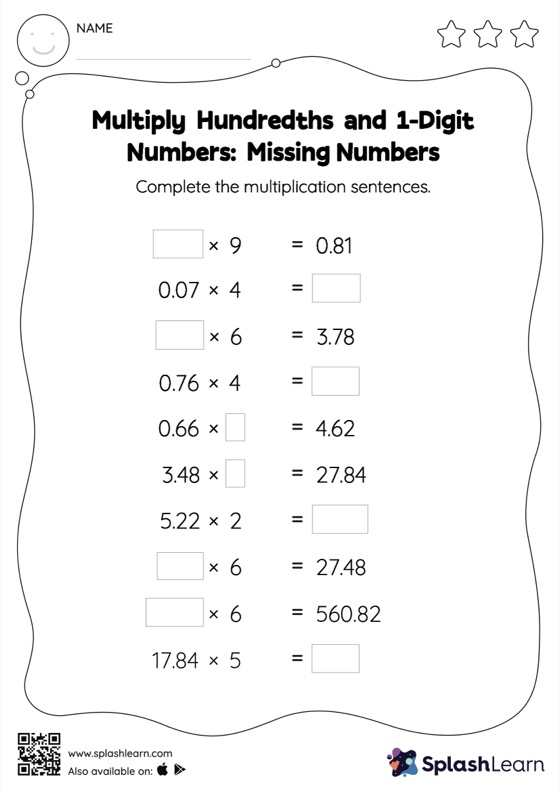# Multiply Hundredths and 1-Digit Numbers: Missing Numbers Worksheet

Home > Multiply Hundredths and 1-Digit Numbers: Missing NumbersWhen multiplying a decimal by a one-digit number, students may ignore the decimal point during calculations and bring it back when writing the result. This multiply hundredths and 1-digit numbers worksheet requires them to find the missing number using this concept. This worksheet is about practicing with the horizontal format in which numbers are written side by side. To develop flexibility with numbers and operations, students need to have enough practice in this format and not just rely on the vertical/column method.